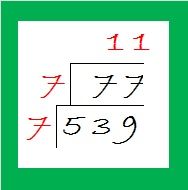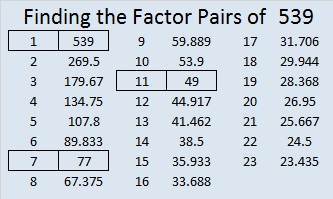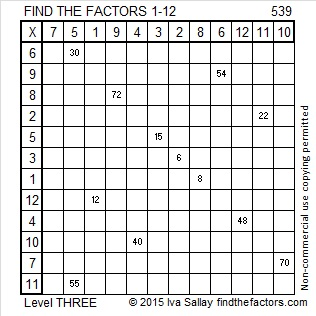# Simplifying √539 and Level 3

If you add up all the prime numbers from 29 to 71, the sum will be 539.

Specifically, 29 + 31 + 37 + 41 + 43 + 47 + 53 + 59 + 61 + 67 + 71 = 539.

How to reduce √539: Since 539 cannot be evenly divided by 100, 4, 9, or 25, look for the smallest prime number that will divide into 539. You will soon note that 539 ÷ 7 = 77. Divide 77 by 7 again to get 11. I like to make a little cake that looks like this:Then I take the square root of everything on the outside of the cake: √539 = (√7)(√7)(√11) = 7√11Print the puzzles or type the solution on this excel file: 12 Factors 2015-06-29

—————————————————————————————————

• 539 is a composite number.
• Prime factorization: 539 = 7 x 7 x 11, which can be written 539 = (7^2) x 11
• The exponents in the prime factorization are 2 and 1. Adding one to each and multiplying we get (2 + 1)(1 + 1) = 3 x 2  = 6. Therefore 539 has exactly 6 factors.
• Factors of 539: 1, 7, 11, 49, 77, 539
• Factor pairs: 539 = 1 x 539, 7 x 77, or 11 x 49
• Taking the factor pair with the largest square number factor, we get √539 = (√49)(√11) = 7√11 ≈ 23.21637353—————————————————————————————————

A Logical Approach to solve a FIND THE FACTORS puzzle: Find the column or row with two clues and find their common factor. Write the corresponding factors in the factor column (1st column) and factor row (top row).  Because this is a level three puzzle, you have now written a factor at the top of the factor column. Continue to work from the top of the factor column to the bottom, finding factors and filling in the factor column and the factor row one cell at a time as you go.